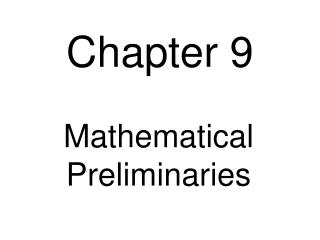DownloadDownload PresentationChapter 9

# Chapter 9

Download Presentation## Chapter 9

- - - - - - - - - - - - - - - - - - - - - - - - - - - E N D - - - - - - - - - - - - - - - - - - - - - - - - - - -
##### Presentation Transcript

1. Chapter 9 Mathematical Preliminaries

2. Stirling’s Approximation Fig. 9.2-1 by trapezoid rule take antilogs . . . . Fig. 9.2-2 by midpoint formula take antilogs  9.2

3. Binomial Bounds Show the volume of a sphere of radius λn in the n-dimensional unit hypercube is: Assuming 0  λ  ½ (since the terms are reflected about n/2) the terms grow monotonically, and bounding the last by Stirling gives: 9.3

4. N.b. 9.3

5. by convention The Gamma Function For n > 1, integrate by parts: dg = e−xdxf = xn−1 Idea: extend the factorial to non-integral arguments. 9.4

6. dr rdθ r dx dy area = rdrdθ area = dxdy 9.4

7. N – Dimensional Euclidean Space Use Pythagorean distance to define spheres: Consequently, their volume depends proportionally on rn converting to polar coordinates 9.5

8. r2 t just verify by substitution 9.5

9. Interesting Facts aboutN-dimensional Euclidean Space Cn→ 0 as n → ∞  Vn(r) → 0 as n → ∞ for a fixed r Volume approaches 0 as the dimension increases! Almost all the volume is near the surface (as n→∞) end of 9.5

10. Angle between the vector (1, 1, …, 1) and each coordinate axis: As n→ ∞: cos θ → 0,  θ → π/2. length of projection along axis What about the angle between random vectors, x and y, of the form (±1, ±1, …, ±1)? length of entire vector For large n, the diagonal line is almost perpendicular to each axis! By definition: Hence, for large n, there are almost 2n random diagonal lines which are almost perpendicular to each other! end of 9.8

11. Chebyshev’s Inequality x2× p(x) ≥ 0 Let X be a discrete or continuous random variable with p(xi) = the probability that X = xi. The mean square is Chebyshev’s inequality 9.7

12. Variance The variance of X is the mean square about the mean value of X, So variance, (via linearity) is: Note: V{1} = 0 → V{c} = 0 & V{cX} = c²V{X} 9.7

13. The Law of Large Numbers Suppose X and Y are independent random variables, with E{X} = a, E{Y} = b, V{X} = σ2, V{Y} = τ2. because of independence Then E{(X− a) ∙ (Y − b)} = E{X − a} ∙ E{Y − b} = 0 ∙ 0 = 0And V{X + Y} = E{(X− a + Y − b)2} = E{(X − a)2} + 2E{(X − a)(Y − b)} + E{(Y − b)2} = V{X} + V{Y} = σ2 + τ2 Consider n independent trials for X; called X1, …, Xn. The expectation of their average is (as expected!): 9.8

14. The variance of their average is (remember independence): So, what is the probability that their average A is not close to the mean E{X} = a? Use Chebyshev’s inequality: Let n→ ∞ Weak Law of Large Numbers: The average of a large enough number of independent trials comes arbitrarily close to the mean with arbitrarily high probability. 9.8